# suranteran

•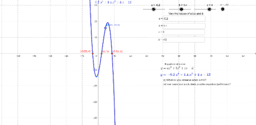### Cubic graphs

Activity

suranteran

•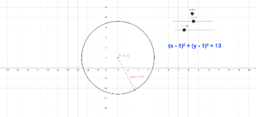### Equation_of_circles

Activity

suranteran

•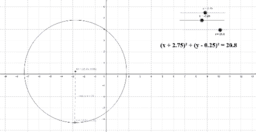### equation of circles

Activity

suranteran

•### graphs of y^2 = kx

Activity

suranteran

•### Intersection of a line and a quadratic curve

Activity

suranteran

•### Quadratic functions and discriminant

Activity

suranteran

•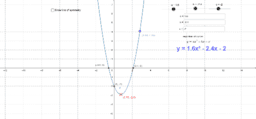### Equation of Quadratic graphs/ Line of symmetry

Activity

suranteran

•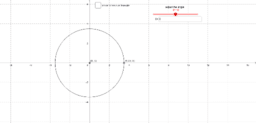### Trigonometric ratio of general angles

Activity

suranteran

•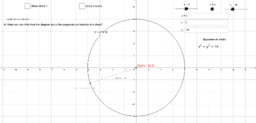### equation of circle

Activity

suranteran

•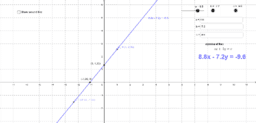### linear line of the form ax+by = c

Activity

suranteran

•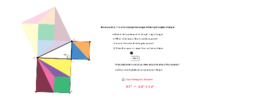### pythagoras theorem

Activity

suranteran

•### trigonometric ratios of acute angle ( sine/ cosine/ tangent)

Activity

suranteran

•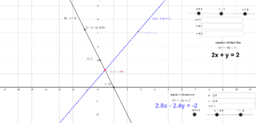### Linear graphs of simultaneous linear equation

Activity

suranteran

•### Linear graphs of simultaneous linear equation

Activity

suranteran

•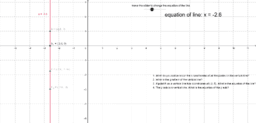### equation of vertical line

Activity

suranteran

•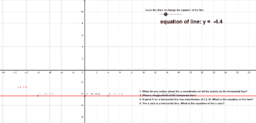Activity

suranteran